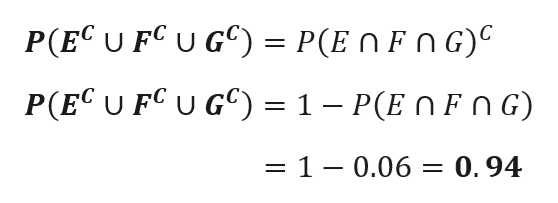# Let E, F and G be three events in S with P(E) = 0.4, P(F) = 0.5, P(G) = 0.29, P(E ∩ F) = 0.28, P(E ∩ G) = 0.11, P(F ∩ G) = 0.14, and P(E ∩ F ∩ G) = 0.06. Find P(EC ∪ FC ∪ GC).

Question
10 views

Let EF and G be three events in S with P(E) = 0.4, P(F) = 0.5, P(G) = 0.29, P(E ∩ F) = 0.28, P(E ∩ G) = 0.11, P(F ∩ G) = 0.14, and P(E ∩ F ∩ G) = 0.06. Find P(EC ∪ FC ∪ GC).

check_circle

Step 1

Given Data

P(E) = 0.4, P(F) = 0.5,P(G) = 0.29, P (E ∩ F) = 0.28, P(E ∩ G) = 0.11, P(F ∩ G) = 0.14, and P(E ∩ F ∩ G) = 0.06.

De-Morgan Law states that

Let E,F and G be three events

Step 2

The required probability is ...help_outlineImage TranscriptioncloseP(EC U FC U G)= P(ENFNG) P(EC U FC U G = 1 - P(E n FnG) 1 - 0.06 = 0.94 fullscreen

### Want to see the full answer?

See Solution

#### Want to see this answer and more?

Solutions are written by subject experts who are available 24/7. Questions are typically answered within 1 hour.*

See Solution
*Response times may vary by subject and question.
Tagged in

### Probability